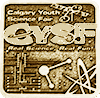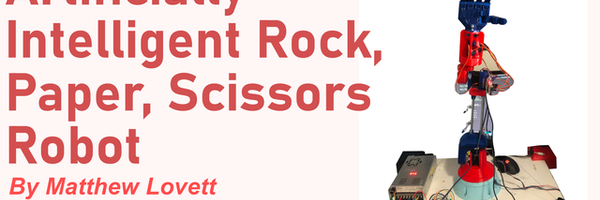GOLD#### Learn to Land

The project uses Deep Q-learning to train a neural network to solve an Open AI gym environment.

### Problem

Given OpenAI gym environments (https://gym.openai.com/envs/#box2d) that transition through various states and have a goal state, train an agent using deep q-learning techniques so that it can move the environment from start to the goal state.

### Method

An OpenAI gym environment (https://gym.openai.com/envs/#classic_control) begins in a start state, transitions through intermediate states and finally reach the terminal state. State transitions are controlled by the agent. The agent tells the environment to take an action and in response the environment transitions to the next state, until the terminal state is reached. Each state transition results in a reward for the agent. 'Lunar Lander' is an example of an OpenAI gym environment in which the goal for the agent is to navigate the lander to its landing pad.

In my research, I found that Reinforcement Learning algorithms are used to train the agent. In particular, for my experiment, I used Q-Learning algorithm. I implemented the algorithm in Python programming language. With the help of my mentor - my father, he translated a very good book on Machine Learning and simplified for me (Hands-On Machine Learning with Scikit-Learn, Keras, and TensorFlow, 2nd Edition by Aurelien Geron) that explains Q-Learning and also has Python code to balance a 'CartPole-v0' OpenAI gym environment. I, however, found that its performance is not very good (more details in Analysis Section). I learned Q-Learning from this book (taught by my father), but implemented all the code on my own. I was able to improve on the performance by using Prioritized Replay Buffer (details below).

The idea behind Q-Learning is for the model to interact with the environment repeatedly and learn the Value of each state. The Value of a state is a number that describes how good it is to be in that state. Once the agent learns the Value of the state, then it can decide whether to visit that state or not. Value of a state is the average of the discounted Returns of that state. A Return of state is the sum of all the discounted future rewards that will be accumulated when visiting that state:

G(t) = R(t+1) + y R(t+2) + y.y R(t + 3) + ... + y.y.y...yR(T)

where G(t) is the value of state at step t, R(t+1) is the reward in state S(t+1) and R(T) is the reward of the terminal state. y is the discount factor (a number less than 1).

In Q-learning, the agent learns estimates of Q-Values in each state. A Q-value describes how good it is to take a specific action in a given state. This is useful for the agent since this allows the agent to choose the best or greedy action in a given state. A series of greedy (most optimal) actions helps the agent navigate the environment towards the goal state. A Q-value function is defined as:

Q(S(t), a) = R(t+1) + yG(t+1)

As described in Reinforcement Learning book (by Sutton and Bartel), in practice, the current estimate of G(t+1) is used. This is known as bootstrapping:

Q(S(t), a) = R(t+1) + y Q(S(t+1), A(t+1))                       (1)

To summarize, the agent learns the Q(S, A) function. This function is represented by a neural network. During training, the neural network is trained using error between its current estimate of Q(S, A) and the target value calculated using Equation (1). This is known as TD (Temporal Difference) error:

TD Error = R(t+1) + y Q(S(t+1), A(t+1)) - Q(S(t), A(t))

The Machine Learning book by Geron implemented a replay buffer to store the SARSA transitions (States, Actions, Next States, Next Rewards). In each training step, a batch of transitions are sampled from this replay buffer. The author suggested using Prioritized Replay Buffer for better performance (Prioritized Experience Replay paper by schaul, johnquan, ioannisa, davidsilver). I implemented this approach and noticed large improvements over Geron's CartPole-v0 implementation (details in Analysis Section).

### Analysis

I was able to train my agent using Q-learning on various OpenAI gym environments and the agent successfully navigated them from start to goal state. Starting point of my implementation was the Python code in Machine Learning Book by Geron, which was taught to me by mentor, my father. I implemented my own algorithm in Python, but improved upon it by using Prioritized Replay Buffer, as suggested in Prioritized Experience Replay paper. I didn't, however, apply weights to transitions sampled from replay buffer as suggested in the paper. I used weight of 1 for all transitions and that seemed to work. My next work would be to see how to apply the weights and their effect.

I also changed my neural network model from what was described in the book. The book created a 2 layer model with 32 nodes each. I created layers with size 1024, 512, 256, 128, 64, and 32. In the book, the author sampled transitions in a batch of 32, but I sampled them 128 transitions at a time.

With these changes, I notced vast improvements to those reported by the author. The graphs below show the differences. The first figure is the Learning Curve in the book for CartPole-v0 OpenAI gym environment (taken from https://nbviewer.jupyter.org/github/ageron/handson-ml2/blob/master/18_reinforcement_learning.ipynb). A Learning Curve plots the total Returns in each interaction with the environment. The maximum possibe in this case is 200. As we can see, the author gets a return of 200 only a few times and maximum number of times, the total return is between 25 and 50. In my case, however, the agent mostly always gets a return of 200.The average return is 150 and the returns start dipping below from 200 after about 320 episodes. For future, I want to investigate if I can improve upon it.

### Conclusion

I implemented Q-Learning for my neural network agent using Python. I used the agent on various OpenAI gym environments (CartPole, MountainCar, and LunarLander), and successfully navigated the environment to goal state (videos in Presentation). I also improved upon the results of CartPole implementation in the Machine Learning book by Geron.

Geron suggested many improvements in his book that I want to try next. One is the use of Double Q-Learning. Also, my implementation is limited to environments with discrete actions. For future work, I would like to learn methods to solve environments with continuous actions. I also want to investigate why my CartPole learning curve (in Analysis Section) dips below maximum reward 200 later and if I can improve it.

For prioritized replay buffer, I used weights of 1. The author suggested using weights for sampled transitions. I want to learn about it and see its affect.

### Acknowledgement

For this project, my mentor was my father. He researched and read through the books and taught me the concepts of Q-learning and Neural Networks. He taught me Python programming concepts and taught me how to design my program. I coded all the various pieces myself.